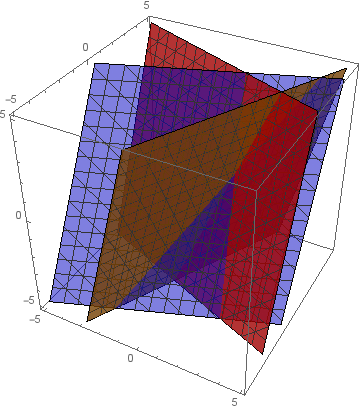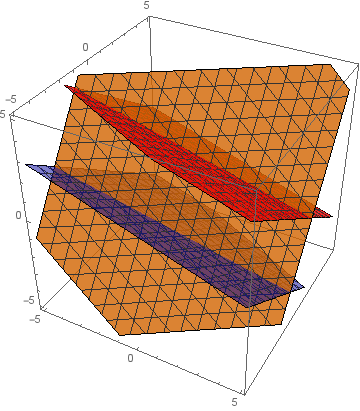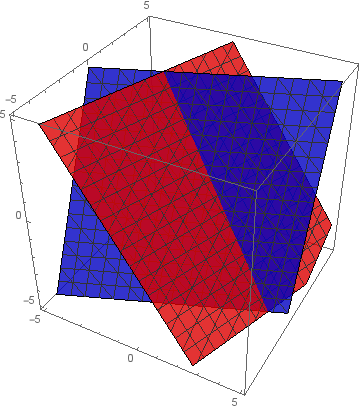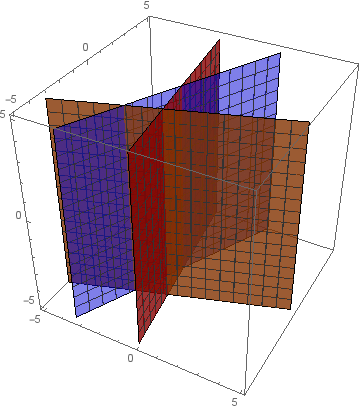### Introduction to Linear Algebra

We’ve now seen how to handle systems of equations in two variables. In this quiz, we’ll take the next logical step forward and attack systems in three variables.

# Three Unknowns

As usual in mathematics, let’s try to reduce the problem to one we already know how to solve. Suppose we have the two equations \begin{aligned} x + 2y + 3z &= 4\\ 3x + 2y + z &= 6. \end{aligned} Which of the following equations is not equivalent to the two equation system?

# Three Unknowns

In the last problem, we saw that given two equations in three unknowns, we can reduce to a single equation with two unknowns (and eliminate whichever of the variables we’d like). Thus, with $3$ equations with $3$ unknowns, we can reduce to two equations in the same two unknowns, which we already know how to handle!

Suppose $x, y, z$ satisfy \begin{aligned} 3x + 2y + z &= 10\\ 4x - 3y + 4z &= 10\\ 5x - 4y + 2z &= 3. \end{aligned} What is $x + y + z?$

# Three Unknowns

Of course, as before it is possible for this method to “fail,” in the sense that the system may no longer have a single solution. For instance, if we have the system \begin{aligned} 3x + 2y + z &= 10\\ 6x + 4y + 2z &= 20\\ 5x - 4y + 2z &= 3. \end{aligned} Then there is no unique solution since the second equation is twice the first (similar to what we saw in the previous quiz). But with $3$ equations, it is not always so easy to detect when this happens, as we see below.

Suppose we have the system \begin{aligned} 3x + 2y + z &= 10\\ 4x - 2y + 4z &= 10\\ 5x + y + az &= 15. \end{aligned} What value of $a$ would lead this system to not have a unique solution?

# Three Unknowns

By now you’ve probably noticed an interesting pattern: to solve a system in two variables, we (usually) need two equations, and to solve a system in three variables, we (usually) need three equations. This leads to a natural question: do we need four equations to solve a system in four variables?

It turns out that the answer is yes: we need at least as many equations as there are degrees of freedom in the system. We’ll come back and formally define this later, but intuitively degrees of freedom correspond to the number of independent variables in the system. For example, if there are no equations in a $3$-variable system, obviously $x, y,$ and $z$ can be anything--so they’re all independent, and there are $3$ degrees of freedom. If there is one equation, then when we pick $x$ and $y,$ the value of $z$ is (usually) forced, so $z$ is no longer independent and there are only $2$ degrees of freedom left. To get rid of all the degrees of freedom — making a unique solution — we need $3$ equations.

# Three Unknowns

In the previous quiz, we saw how $2$-variable equations can be represented by lines, and solving a system of equations can be viewed as finding the intersection of these lines. Similarly, a $3$-variable equation can be viewed as a plane, and solving a $3$-variable system can be viewed as finding the intersection of these planes.

This also shows why there are more “exceptions,” or degenerate systems, to the general rule of $3$ equations being enough for $3$ variables. Which of the following images of planes does not correspond to a degenerate system of equations?

A)B)C)D)×